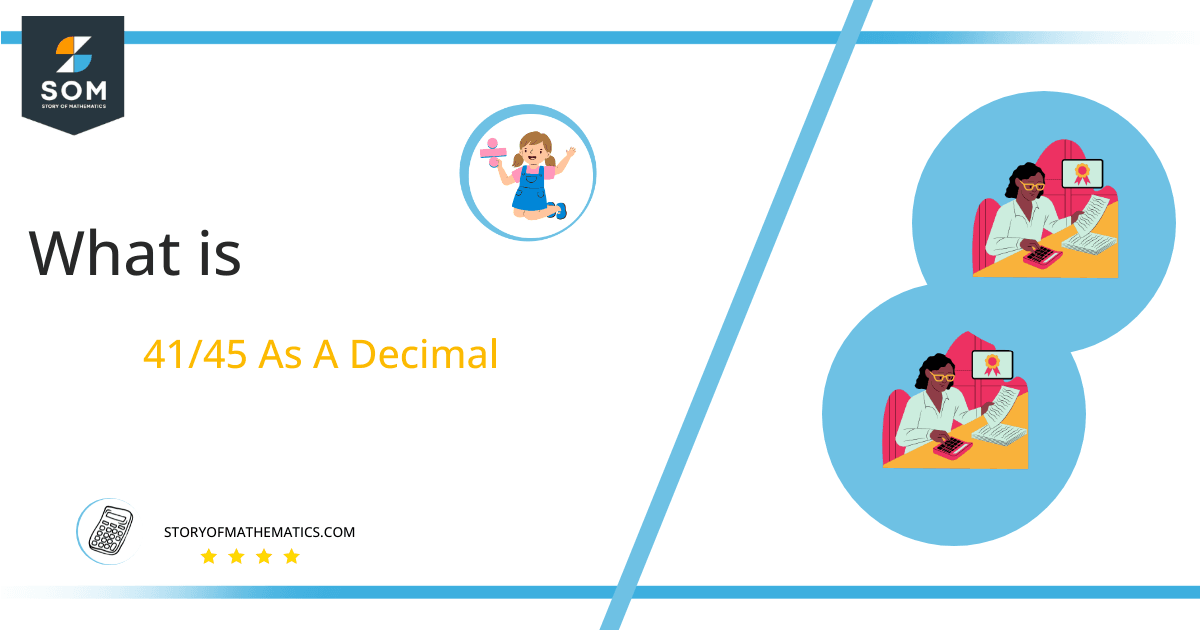# What Is 41/45 as a Decimal + Solution With Free Steps

The fraction 41/45 as a decimal is equal to 0.911.

The basic arithmetic operation of division is often expressed in the form of a fraction p/q, which is both compact and easier to write. For all purposes, the fraction notation is mathematically equivalent to the usual p $\boldsymbol\div$ q. The only difference is that p and q are now called the numerator and denominator.Here, we are more interested in the division types that result in a Decimal value, as this can be expressed as a Fraction. We see fractions as a way of showing two numbers having the operation of Division between them that result in a value that lies between two Integers.

Now, we introduce the method used to solve said fraction to decimal conversion, called Long Division, which we will discuss in detail moving forward. So, let’s go through the Solution of fraction 41/45.

## Solution

First, we convert the fraction components, i.e., the numerator and the denominator, and transform them into the division constituents, i.e., the Dividend and the Divisor, respectively.

This can be done as follows:

Dividend = 41

Divisor = 45

Now, we introduce the most important quantity in our division process: the Quotient. The value represents the Solution to our division and can be expressed as having the following relationship with the Division constituents:

Quotient = Dividend $\div$ Divisor = 41 $\div$ 45

This is when we go through the Long Division solution to our problem.Figure 1

## 41/45 Long Division Method

We start solving a problem using the Long Division Method by first taking apart the division’s components and comparing them. As we have 41 and 45, we can see how 41 is Smaller than 45, and to solve this division, we require that 41 be Bigger than 45.

This is done by multiplying the dividend by 10 and checking whether it is bigger than the divisor or not. If so, we calculate the Multiple of the divisor closest to the dividend and subtract it from the Dividend. This produces the Remainder, which we then use as the dividend later.

Now, we begin solving for our dividend 41, which after getting multiplied by 10 becomes 410.

We take this 410 and divide it by 45; this can be done as follows:

410 $\div$ 45 $\approx$ 9

Where:

45 x 9 = 405

This will lead to the generation of a Remainder equal to 410 – 405 = 5. Now this means we have to repeat the process by Converting the 5 into 50 and solving for that:

50 $\div$ 45 $\approx$ 1

Where:

45 x 1 = 45

This, therefore, produces another Remainder which is equal to 50 – 45 = 5. Now we must solve this problem to Third Decimal Place for accuracy, so we repeat the process with dividend 50.

50 $\div$ 45 $\approx$ 1

Where:

45 x 1 = 45

Finally, we have a Quotient generated after combining the three pieces of it as 0.911, with a Remainder equal to 5.Images/mathematical drawings are created with GeoGebra.